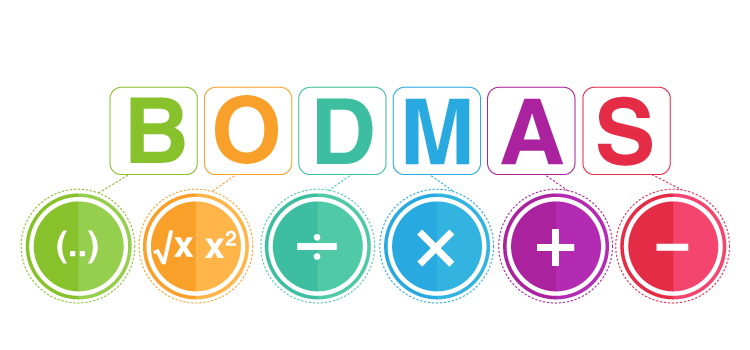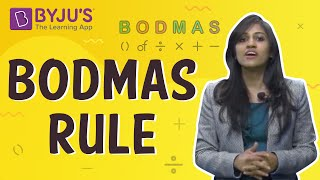A number is a mathematical value used to count and measure different objects. With the help of the numbers we all are able to add, subtract, divide and multiple. Here we will be learning how to compare numbers, expand the number and also learn about the largest and the smallest numbers.

Roman numerals and the Hindu-Arabic numeral system are the two different types of number system used for writing numbers in many places. We all can see the roman numerals in clocks, page numbers, school timetable in syllabus page, etc.

## Introduction

### Introduction to numbers

• Numbers are arithmetic values.
• Numbers are used to convey the magnitude of everything around us.

### Comparing numbers

Comparing numbers when the total number of digits is different

• The number with most number of digits is the largest number by magnitude and the number with least number of digits is the smallest number.
Example: Consider numbers: 22, 123, 9, 345, 3005. The largest number is 3005 (4 digits) and the smallest number is 9 (only 1 digit)

Comparing numbers when the total number of digits is same

• The number with highest leftmost digit is the largest number. If this digit also happens to be the same, we look at the next leftmost digit and so on.
Example: 340, 347, 560, 280, 265. The largest number is 560 (leftmost digit is 5) and the smallest number is 265 (on comparing 265 and 280, 6 is less than 8).

## Ascending and Descending Order and Shifting Digits

### Ascending order and Descending order

• Ascending Order: Arranging numbers from the smallest to the greatest.
• Descending Order: Arranging numbers from the greatest to the smallest number.
• Example: Consider a group of numbers: 32, 12, 90, 433, 9999 and 109020.
They can be arranged in descending order as 109020, 9999, 433, 90, 32 and 12, and in ascending order as 12, 32, 90, 433, 9999 and 109020.

To know more about Ascending Order, visit here.
To know more about descending Order, visit here.

### How many numbers can be formed using a certain number of digits?

• If a certain number of digits are given, we can make different numbers having the same number of digits by interchanging positions of digits.
• Example: Consider 4 digits: 3, 0, 9, 6. Using these four digits,
(i) Largest number possible = 9630
(ii) Smallest number possible = 3069 (Since 4 digit number cannot have 0 as the leftmost number, as the number then will become a 3 digit number)

### Shifting digits

• Changing the position of digits in a number, changes magnitude of the number.
• Example: Consider a number 789. If we swap the hundredths place digit with the digit at units place, we will get 987 which is greater than 789.
Similarly, if we exchange the tenths place with the units place, we get 798, which is greater than 789.

### Place value

• Each place in a number, has a value of 10 times the place to its right.
• Example: Consider number 789.
(i) Place value of 7 = 700
(ii) Place value of 8 = 80
(iii) Place value of 9 = 9

To know more about Place Value, visit here

## Larger Numbers and Estimates

### Introducing large numbers

Large numbers can be easily represented using the place value. It goes in the ascending order as shown below

 8 digits 7 digits 6 digits 5 digits 4 digits 3 digits 10 million (1 crore) 1 million (10 lakhs) Hundred Thousands (1 lakh) Ten Thousands Thousands Hundreds
• Largest 3 digit number + 1 = Smallest 4 digit number.
• Largest 4 digit number + 1 = Smallest 5 digit number, and so on.
Example: 9999 (greatest 4 digit number) + 1 = 10,000 (smallest 5 digit number)
• We can convert every large numbers in terms of smaller numbers:
Remember, 1 hundred = 10 tens
1 thousand = 10 hundreds     = 100 tens
1 lakh         = 100 thousands  = 1000 thousands
1 crore       = 100 lakhs          = 10,000 thousands

To know more about “Introduction of Large Number”, visit here

### Estimation

• When there is a very large figure, we approximate that number to the nearest plausible value. This is called estimation.
• Estimating depends on the degree of accuracy required and how quickly the estimate is needed.
• Example:
 Given Number Appropriate to Nearest Rounded Form 75847 Tens 75850 75847 Hundreds 75800 75847 Thousands 76000 75847 Tenth thousands 80000

To know more about “Estimation of Numbers”, visit here

### Estimating sum or difference

• Estimations are used in adding and subtracting numbers.
• Example of estimation in addition: Estimate 7890 + 437.
Here 7890 > 437.
Therefore, round off to hundreds.
7890 is rounded off to       7900
437 is rounded off to      +   400
Estimated Sum =              8300
Actual Sum       =              8327
• Example of estimation in subtraction: Estimate 5678 – 1090.
Here 5678 > 1090.
Therefore, round off to thousands.
5678 is rounded off to       6000
1090 is rounded off to    – 1000
Estimated Difference =     5000
Actual Difference       =     4588

### Estimating products of numbers

• Round off each factor to its greatest place, then multiply the rounded off factors.
• Estimating the product of 199 and 31:
199 is rounded off to 200
31 is rounded off to 30
Estimated Product = 200 × 30 = 6000
Actual Result = 199 × 31 = 6169

## BODMAS

### BODMAS

• We follow an order to carry out mathematical operations. It is called as BODMAS rule.While solving mathematical expressions, parts inside a bracket are always done first, followed by of, then division, and so on.

• Example :

[(5 + 1) × 2] ÷ (2 × 3) + 2 – 2 = ?

[(5 + 1) × 2] ÷ (2 × 2) + 2 – 2….{Solve everything which is inside the brackets}

= [6 × 2] ÷ 6 + 2 – 2…..{Multiplication inside brackets}

= 12 ÷ 6 + 2 – 2……{Division}

= 2 + 2 – 2……{Addition}

= 4 – 2…….{Subtraction}

= 2

### Using brackets

1. Using brackets can simplify mathematical calculations.
2. Example :
• 7 × 109 = 7 × (100 + 9) = 7 × 100 + 7 × 9 = 700 + 63 = 763
• 7 × 100 + 6 × 100 = 100 × (7 + 6) = 100 × 13 = 1300To know more about “BODMAS”, visit here.

### Roman Numerals

• Digits 09 in Roman are represented as : I, II, III, IV, V, VI, VII, VIII, IX, X
• Some other Roman numbers are : I = 1, V = 5 , X = 10 , L = 50 , C = 100 , D = 500 , M = 1000

Rules for writing Roman numerals

• If a symbol is repeated, its value is added as many times as it occurs.
Example: XX = 10 + 10 = 20
• A symbol is not repeated more than three times. But the symbols X, L and D are never repeated.
• If a symbol of smaller value is written to the right of a symbol of greater value, its value gets added to the value of greater symbol.
Example: VII = 5 + 2 = 7
• If a symbol of smaller value is written to the left of a symbol of greater value, its value is subtracted from the value of greater symbol.
Example: IX = 10 – 1 = 9.
• Some examples : 105 = CV , 73 = LXXIII and 192 = 100 + 90 + 2 = C  XC  II = CXCIITo know more about “Roman Numerals”, visit here.

## Frequently asked Questions on CBSE Class 6 Maths Notes Chapter 1: Knowing Our Numbers

### What is ‘BODMAS’?

The BODMAS acronym stands for brackets, orders, division, multiplication, addition, subtraction.

### What are ‘Roman numerals’?

Roman numerals are the symbolic representation of numbers that do not follow a place value system.

### What are ‘Real numbers’?

Real numbers are numbers that include both rational and irrational numbers.